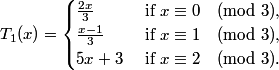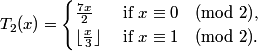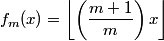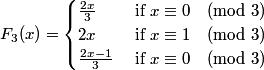### Mapping T1

The iterates y, T1(y), T1(T1(y)),... of the functionare printed and the number of steps taken to reach one of the integers 0, -1, -4, -19 is recorded.

Benoit Cloitre in an email to Jeff Lagarias dated July 19, 2011, has conjectured that every trajectory starting from a positive integer will eventually reach 0.
It is also clear experimentally that any trajectory starting from a negative integer will eventually reach one of three cycles beginning with -1, -4 or -19.

Cycles: (i) 0,0; (ii) -1,-2,-1; (iii) -4,-17,-6,-4; (iv) -19,-92,-31,-152,-51,-34,-167,-56,-19.

Heuristics: Visit Generalized 3x+1 functions and Markov matrices, where we find, with d = 3 and m0=2, m1 = 1, m2 = 15 and x0=0, x1 = 0, x2 = 3,

```    Q(3) = 1/3  1/3 1/3
1/3  1/3 1/3
0    1   0
```
has stationary vector (1/4, 1/2, 1/4). Also

(2/3)1/4(1/3)1/2(15/3)1/4 < 1.

Consequently we expect all trajectories to eventually cycle.

### Mapping T2.

Benoit Cloitre in an email to Jeff Lagarias dated July 20, 2011, has conjectured that every trajectory starting from a positive integer will eventually reach 0 or 2.
It is also clear experimentally that any trajectory starting from a negative integer will eventually reach -1.

Cycles: (i) 0,0; (ii) 2,7,2: (iii) -1,-1.

Heuristics: This function can be regarded as a six-branched mapping. Accordingly, visit Generalized 3x+1 functions and Markov matrices, where we find,
with d = 6 and m0 = 21, m1 = 2, m2 = 21, m3 = 2, m4 = 21, m5 = 2 and x0 = 0 = x1 = x2 = x3 = x4 = x5, that

```Q(6) =
1/2 	 0    0   1/2 	0    0
1/3 	 0   1/3   0   1/3   0
0 	1/2   0    0   1/2   0
0 	1/3   0   1/3 	0   1/3
0 	 0   1/2   0 	0   1/2
0 	1/3   0   1/3 	0   1/3
```
This Markov matrix has stationary vector (2/15,3/15,2/15,3/15,2/15,3/15) and the weighted product (21/6)2/15(2/6)3/15 ··· is less than 1, and this allows us to predict that all trajectories will eventually cycle.

### Mapping T3.

For this mapping, Benoit Cloitre has conjectured that every trajectory starting from a positive integer will eventually reach 0, 4, 6 or 24.
It is also clear experimentally that any trajectory starting from a negative integer will eventually reach -1.

Cycles: (i) 0,0; (ii) 4,13,4: (iii) 6,19,6 (iv) 24, 78,...,73,24 (length 87) (v) -1,-1.

Heuristics: This function can be regarded as a twelve-branched mapping and an analysis of the 12x12 Markov matrix Q(12) suggests that all trajectories will eventually cycle.

### Mapping Fm

First fm(x) is defined by.

Then.

For m = 3, 5 and m ≥ 7, Benoit Cloitre conjectures that the trajectories of Fm will eventually enter one of finitely many cycles.
This mapping can be regarded as a 2m-branched generalized 3x+1 mapping if m is even, and an m-branched mapping if m is odd. See the BC program cloitrem in which function(m) prints Fm(x) in two ways. For example,Here all trajectories appear to enter one of six cycles (i) 0,0 (ii) -1,-1 (iii) 1,2,1 (iv) -2,-3,-4,-2 (v) -5,-10,-7 (vi) 19,38,25,50,33,22,44,29,19.

We can use the Markov matrix approach of generalized 3x+1 functions to predict the behaviour of trajectories. See manuscript.

We find for example, with m = 3, m0=2, m1 = 6, m2 = 2 and x0 = 0= x1 = x2,

```    Q(3) = 1/3  1/3 1/3
0    0   1
1/3  1/3 1/3
```
which has stationary vector (1/4, 1/4, 1/2). Also

(2/3)1/4(6/3)1/4(2/3)1/2 < 1.

Consequently we expect all trajectories to eventually cycle.

A list of cycles found for 2 ≤ m ≤ 2000 is also attached.
The examples of F29 and F153 are striking, as there are apparently 165 and 416 cycles, respectively.

Enter y:

Mapping T1
Mapping T2
Mapping T3
Mapping F3
Mapping F29